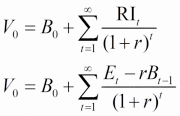# Cost of equity -> Market Value or Book Value?

Hi everyone,

I know when calculating the WACC for a DCF model usually the cost of equity (in %) is multiplied with the market value of equity, not the book value, right? But when valuing a stock with the residual income model, usually the cost of equity (in %) is multiplied with the book value of equity. Here is the equation for the residual income model (same as in the image)…

Value (today) = Book Value (totay) + (Earnings for period 1 - (cost of equity x book value of equity)

Can you please tell me, why we use book value of equity here instead of market value, which I think makes more sense? Thank you!It is the (fair) market value of equity that you are trying to compute, given the book value of equity.

That is what V(0) is in the equation (well market value of equity per share).

I think its becuase you are trying to find the intrinsic value to determine if the market value is over/undervalued. So you use Book Value and apply residual income to add the future value of growth discounted to the present period. Market Value takes future earnings into account so using Market value and adding the residual income equation would be redundant.

You would use Market Value for WACC becuase that is a actual current representation of cost of equity, not the intrinsic or perceived cost of equity. Make sense?